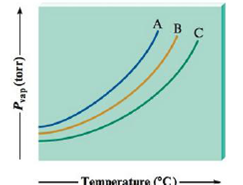# Consider the following vapor pressure versus temperature plot for three different substances: A, B, and C. If the three substances are CH 4 , SiH 4 , and NH 3 , match each curve to the correct substance.### Chemistry: An Atoms First Approach

2nd Edition
Steven S. Zumdahl + 1 other
Publisher: Cengage Learning
ISBN: 9781305079243

#### Solutions

Chapter
Section### Chemistry: An Atoms First Approach

2nd Edition
Steven S. Zumdahl + 1 other
Publisher: Cengage Learning
ISBN: 9781305079243
Chapter 9, Problem 111AE
Textbook Problem
5 views

## Consider the following vapor pressure versus temperature plot for three different substances: A, B, and C.If the three substances are CH4, SiH4, and NH3, match each curve to the correct substance.

Interpretation Introduction

Interpretation: In plot, given substance to be matched with corresponding curve.

Concept introduction

At given temperature, the vapor is elevated by pressure in thermodynamic equilibrium with its solid or liquid phase.

### Explanation of Solution

Explanation

Record the given data

The plot is recorded as shown.

To explain the compounds which are matched with corresponding curves.

At any temperature, the plot shows that compound A has a high vapour pressure than compound B, with compound C having the lowest vapor pressure. Therefore, the sub compound with the weakest intermolecular forces is A, and the compound with the strongest intermolecular forces is C.

NH3 could form hydrogen-bonding interactions, whereas the others cannot

### Still sussing out bartleby?

Check out a sample textbook solution.

See a sample solution

#### The Solution to Your Study Problems

Bartleby provides explanations to thousands of textbook problems written by our experts, many with advanced degrees!

Get Started

Find more solutions based on key concepts
The following are complementary proteins: pot roast and chicken pot roast and carrots rice and French fries pea...

Nutrition: Concepts and Controversies - Standalone book (MindTap Course List)

Unlike mass, weight is not a(n) ___ property. (1.4)

An Introduction to Physical Science

Name the products of the following transamination reaction:

Introduction to General, Organic and Biochemistry

2.53 What is meant by the phrase organic chemistry?

Chemistry for Engineering Students

Review. A student performs a ballistic pendulum experiment using an apparatus similar to that discussed in Exam...

Physics for Scientists and Engineers, Technology Update (No access codes included)

a 7.11 hg to g, b 5.27 x 107 m to pm, c 3.63 x 106 g to dag.

Introductory Chemistry: An Active Learning Approach